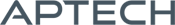# Loglinear Analysis MT

Loglinear Analysis MT

Availability: Out of stock

The Loglinear Analysis MT application module (LOGLIN) contains procedures for the analysis of categorical data using loglinear analysis. This application is thread-safe and takes advantage of structures. The estimation is based on the assumption that...
Overview

## Loglinear Analysis MT

The Loglinear Analysis MT application module (LOGLIN) contains procedures for the analysis of categorical data using loglinear analysis. This application is thread-safe and takes advantage of structures.

The estimation is based on the assumption that the cells of the K-way table are independent Poisson random variables. The parameters are found by applying the Newton-Raphson method using an algorithm found in A. Agresti (1984) Analysis of Ordinal Categorical Data.

You may construct your own design matrix or use LOGLIN procedures to compute one for you. You may also select the type of constraint and the parameters.

Platform: Windows, Mac and Linux.

Requirements: GAUSS/GAUSS Engine/GAUSS Light v8.0 or higher.

Features

Features

• Fits a hierarchical model given fit configurations
• Will fit all 3-way hierarchical models of a table
• Provides for cell weights
• LOGLIN can estimate most of the models described in such texts as Y.M.M. Bishop, S.E. Fienberg, and P.W. Holland (1975) Discrete Multivariate Analysis, S. Haberman (1979) Analysis of Qualitative Data, Vols. 1 and 2, as well as the book by A. Agresti.

Product Inquiry

5 + 4 = enter the result ex:(3 + 2 = 5)

﻿